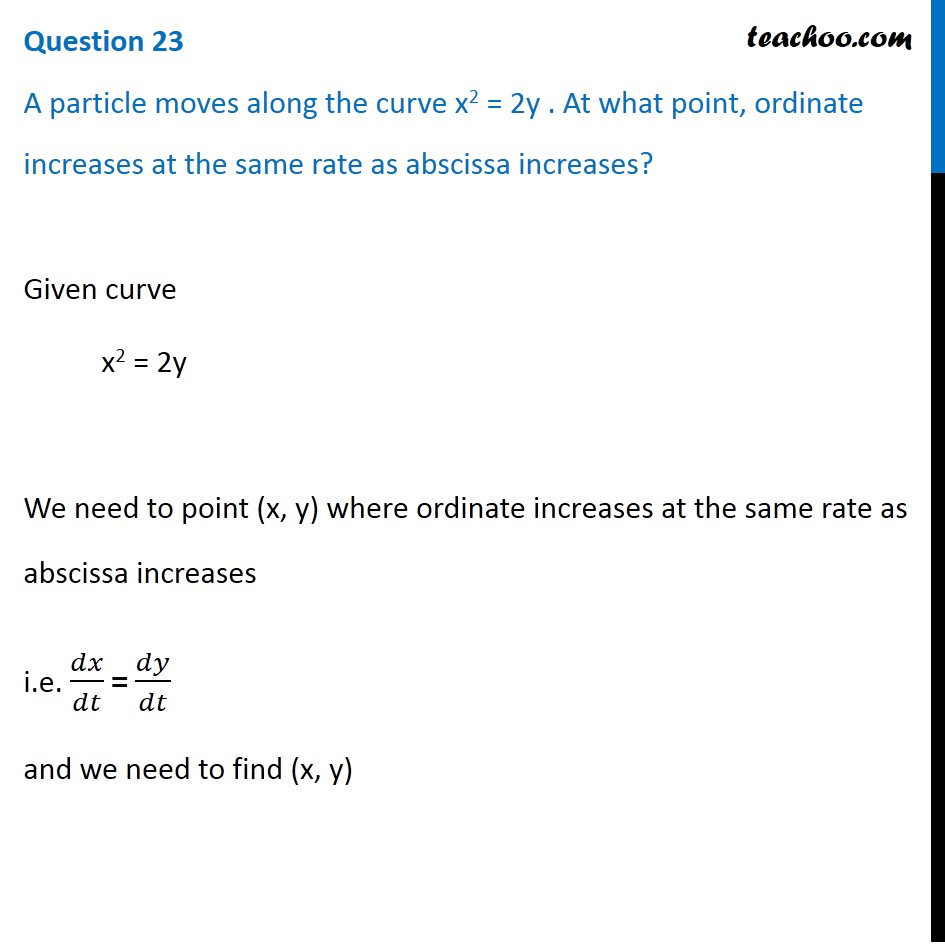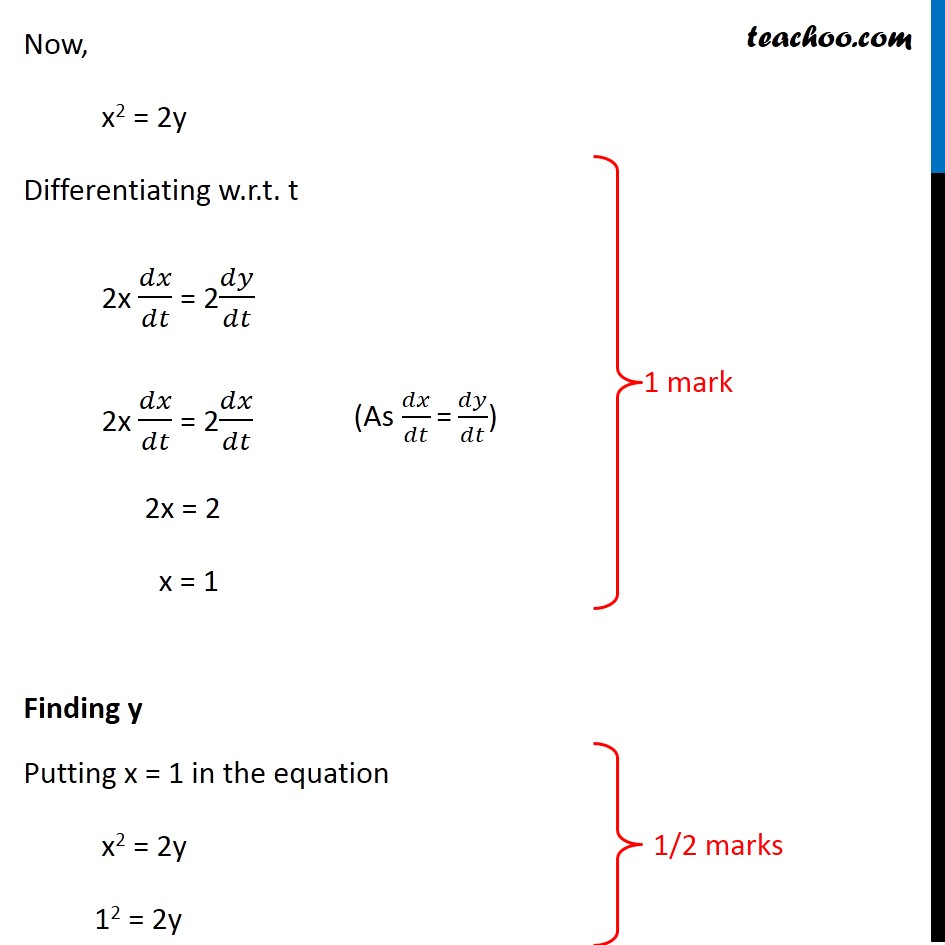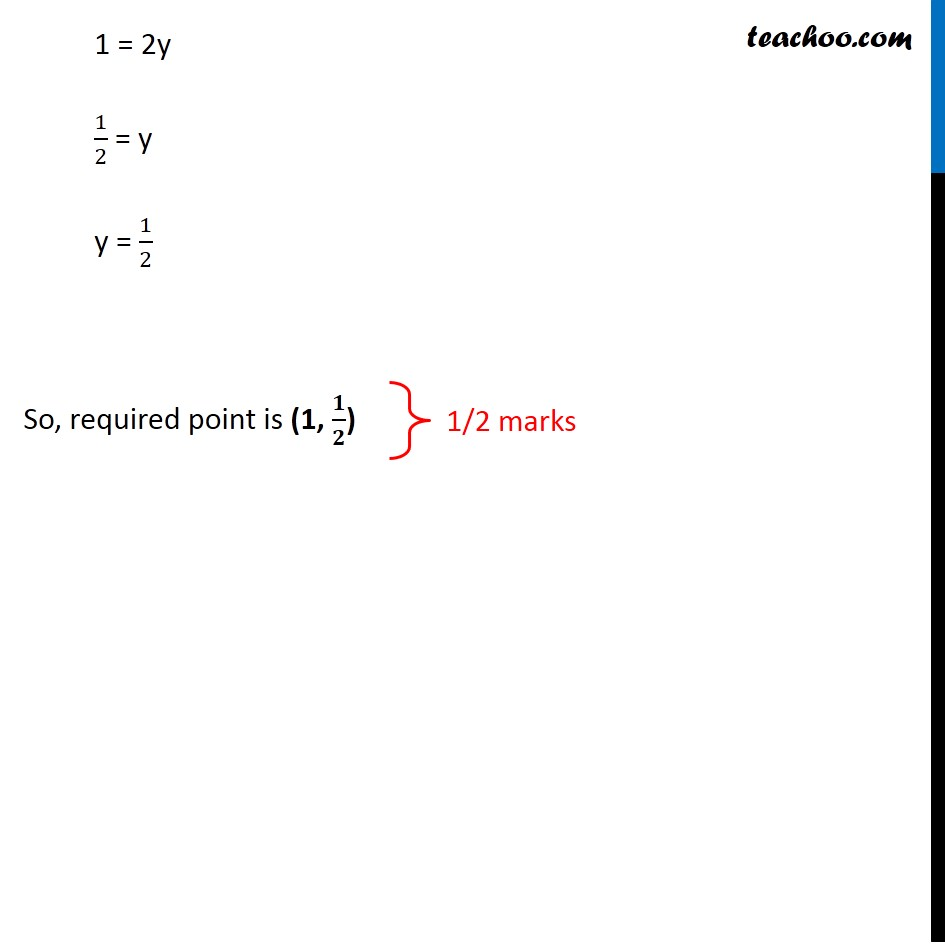CBSE Class 12 Sample Paper for 2020 Boards

Class 12
Solutions of Sample Papers and Past Year Papers - for Class 12 Boards

## increases at the same rate as abscissa increases?Learn in your speed, with individual attention - Teachoo Maths 1-on-1 Class

### Transcript

Question 23 A particle moves along the curve x2 = 2y . At what point, ordinate increases at the same rate as abscissa increases? Given curve x2 = 2y We need to point (x, y) where ordinate increases at the same rate as abscissa increases i.e. 𝑑𝑥/𝑑𝑡 "=" 𝑑𝑦/𝑑𝑡 and we need to find (x, y) Now, x2 = 2y Differentiating w.r.t. t 2x 𝑑𝑥/𝑑𝑡 = 2𝑑𝑦/𝑑𝑡 2x 𝑑𝑥/𝑑𝑡 = 2𝑑𝑥/𝑑𝑡 2x = 2 x = 1 Finding y Putting x = 1 in the equation x2 = 2y 12 = 2y (As 𝑑𝑥/𝑑𝑡 "=" 𝑑𝑦/𝑑𝑡) 1 = 2y 1/2 = y y = 1/2 So, required point is (1, 𝟏/𝟐)